#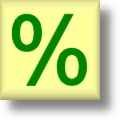Percent Worksheets

## Percent Worksheets for Practice

Here is a graphic preview for all of the percent worksheets. You can select different variables to customize these percent worksheets for your needs. The percent worksheets are randomly created and will never repeat so you have an endless supply of quality percent worksheets to use in the classroom or at home. Our percent worksheets are free to download, easy to use, and very flexible.

These percent worksheets are a great resource for children in Kindergarten, 1st Grade, 2nd Grade, 3rd Grade, 4th Grade, and 5th Grade.

Click here for a Detailed Description of all the Percent Worksheets.

If you're looking for a great tool for converting fraction to percent or percent to fraction check out this online Percentage Calculator.

## Quick Link for All Percent Worksheets

Click the image to be taken to that Percent Worksheet.

#### Table of Common PercentsPercent Worksheets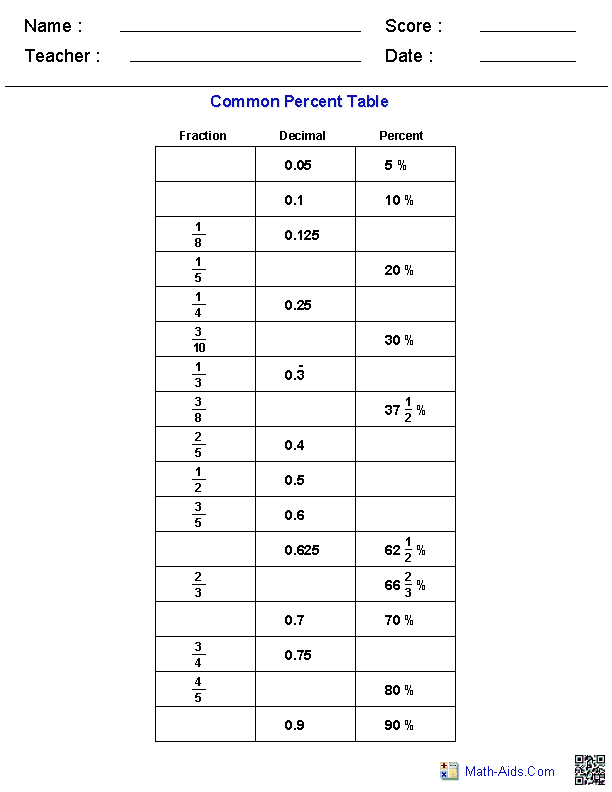#### Multiplying Percents that are Powers of TenPercent Worksheets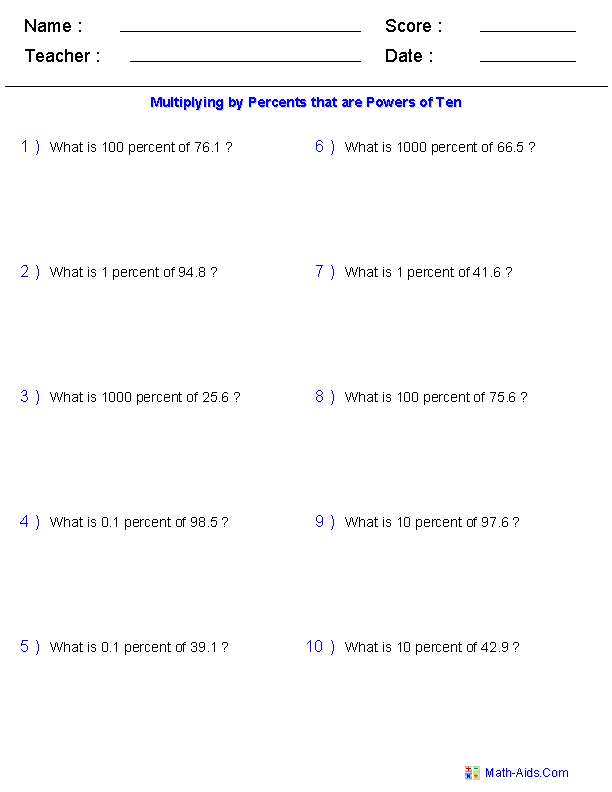#### Percentage CalculationsPercent Worksheets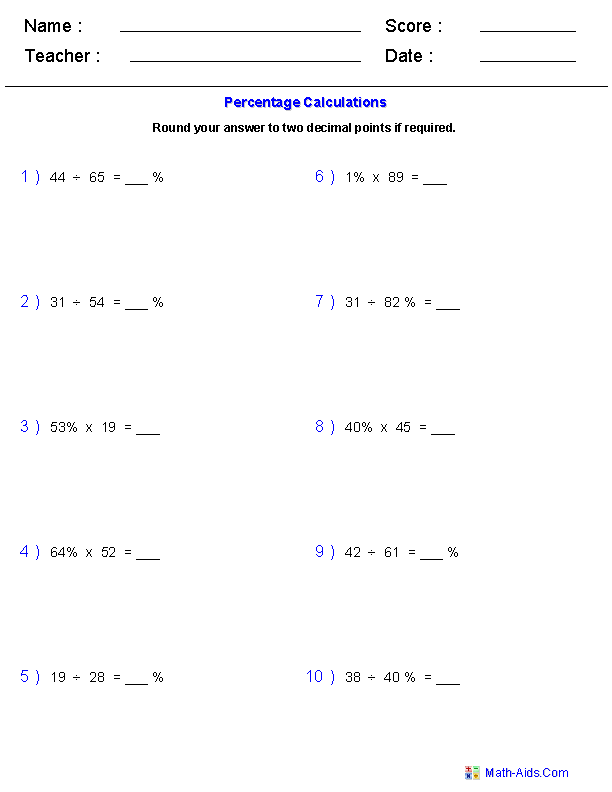#### Converting Between Percents,Decimals, and Fractions Worksheets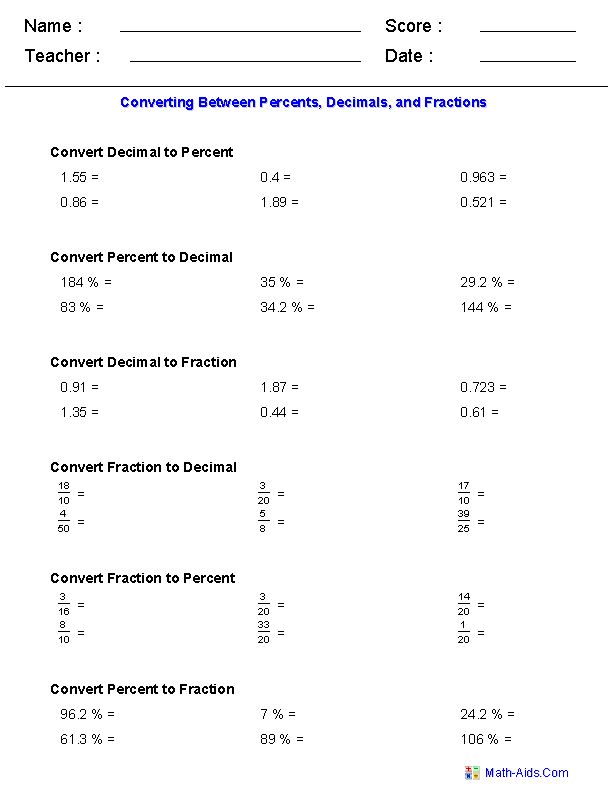Recommended Videos

## Detailed Description for All Percent Worksheets

Table of Common Percents
Percent Worksheets

These percent worksheets are great for learning commonly recognized percents and their fraction and decimal equivalents. These percent worksheets will produce a table of equivalent percents for the student to work with. You may select to leave some blank or completely fill out the table.

Multiplying by Percents that are Powers of Ten
Percent Worksheets

These percent worksheets are great for practicing multiplying by percents that are powers of ten. You may select from 1%, 10%, 100%, 1000%, or .01% to use in the problems. You may select the range of numbers to work with as well as whole number or decimal numbers. You may vary the format of the problems between numerical or word problems. These percent worksheets will produce ten problems per worksheet.

Percentage Calculations
Percent Worksheets

These percent worksheets are great for practicing percentage calculations. You may select three different types of problems and have the problems be in either numerical or word formats. You may select the range of numbers to work with as well as whole number or decimal numbers. You may specify how many decimal points to round the answers. These percent worksheets will produce ten problems per worksheet.

Converting Between Percents,
Decimals, and Fractions Worksheets

These percent worksheets are great for practicing converting between percents, decimals, and fractions. You may select six different types of percentage conversion problems with three different types of numbers to convert. These percent worksheets will produce 30 or 36 problems per worksheet depending on your selection.